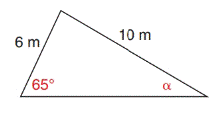Chapter 11.4, Problem 18EElementary Geometry For College St...

7th Edition
Alexander + 2 others
ISBN: 9781337614085

Solutions

Chapter
SectionElementary Geometry For College St...

7th Edition
Alexander + 2 others
ISBN: 9781337614085
Textbook Problem

In Exercises 17 to 22, use a form of the Law of Sines to find the measure of the indicated side or angle. Angle measures should be found to the nearest degree and lengths of sides to the nearest tenth of a unit.To determine

To find:

The angle of the given triangle, by using the Law of Sines.

Explanation

Formula:

The Law of Sines,

sinαa=sinβb=sinγc

Where α, β, and γ is the acute angle of the triangle, and a, b, and c is the sides of the triangle.

Calculation:

Given:

Here, take a=6 m

c=10 m

γ=65°

Find α:

By the Law of Sines,

sinαa=sinβb=sinγc

Take,

sinαa=sinγc

Here, a=6 m

c=<

Still sussing out bartleby?

Check out a sample textbook solution.

See a sample solution

The Solution to Your Study Problems

Bartleby provides explanations to thousands of textbook problems written by our experts, many with advanced degrees!

Get Started

Simplify: 2025

Elementary Technical Mathematics

In problems 63-73, factor each expression completely. 66.

Mathematical Applications for the Management, Life, and Social Sciences

In Exercises 13-20, sketch a set of coordinate axes and plot each point. 19. (4.5, 4.5)

Applied Calculus for the Managerial, Life, and Social Sciences: A Brief Approach

Differentiate the function. g(t)=1+lnt

Single Variable Calculus: Early Transcendentals, Volume I

For y = sin2 x + cos2 x, y = _____. a) 2 sin x 2 cos x b) 2 sin x cos x c) 4 sin x cos x d) 0

Study Guide for Stewart's Single Variable Calculus: Early Transcendentals, 8th

In Exercises 724, compute the indicated products. 

Finite Mathematics for the Managerial, Life, and Social Sciences